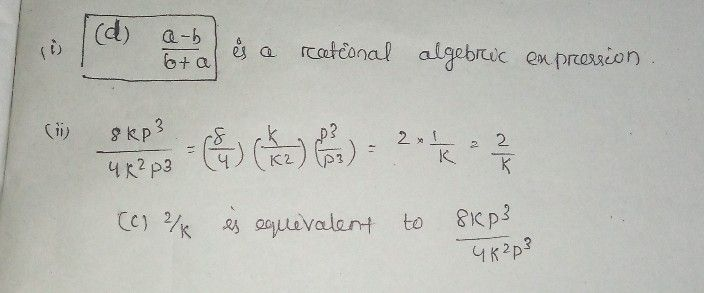Symbol
ProblemIDENTIFICATION aaqqqqqqqqqqqqqqqqqqq $1.1t$ is a ratio of two polynomials provided that the denomina 2. It is a comparison of two or more numbers that indica contains another. $3$ $ln$ the given expression, identify the simplest form : $4.Tn$ mathematical expression of " the cube of a number $5$ $A$ term which is a factor found in the numerator and att elect the correct answer, write your answer in your Activity notebook / Ma Which of the following expressions is a rational algebraic expression? a. $\dfrac {x} {\sqrt{3y} }$ $b$ $\dfrac {3c^{-3}} {\sqrt{\left(a+1\right)} }$ $c.4y^{-2}+z^{-3}$ $d$ $\dfrac {a-b} {b+a}$ What is the rational algebraic expression equivalent to $\dfrac {\left(8kp^{3}\right)} {4k^{2}p^{3}}7$ $a.4k^{2}p^{0}$ b. $2k^{2}p^{3}$ $G$ $\dfrac {2} {k}$ d. $2k^{-1}$
7th-9th grade
Algebra
Search count: 114
SolutionQanda teacher - priyamamun